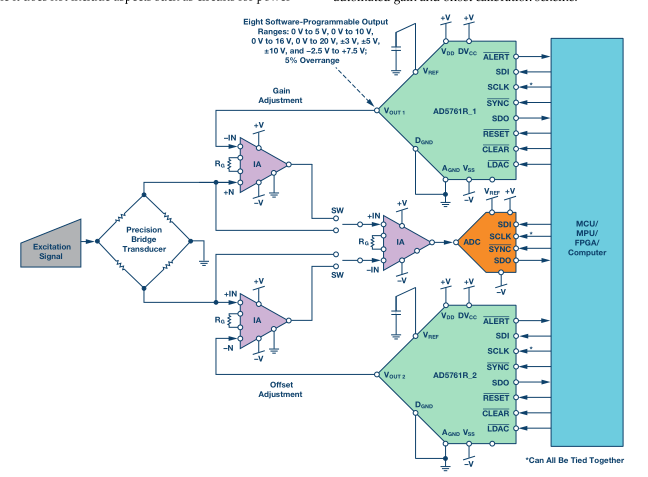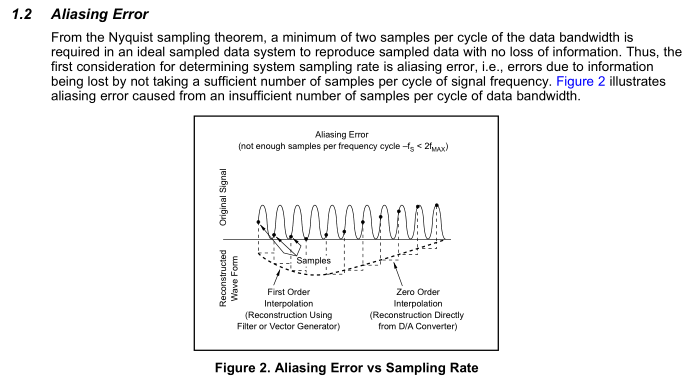If you have a related question, please click the "Ask a related question" button in the top right corner. The newly created question will be automatically linked to this question.

# DAC7760: How to match high speed ADC and lower speed DAC

Part Number: DAC7760
Other Parts Discussed in Thread: DAC7822, TIDA-00170, TIDA-00119, DAC8760

In order to fit input signal frequency I have to select the higher speed ADC, on the other hand have to select the precision DAC to satisfy the accuracy. How do the ADC handle the input signal when the DAC has not finished converting? The MCU processea the convered data. Does the ADC repeat converting and wait for the DAC? Are there any better suoltions?

• Hi Jingtai,

Depending on how fast the ADC is sampling, you could potentially measure the DAC while is slewing between to output codes.  If you are trying to avoid this you could synchronize the ADC to sample after the DAC has settled, using the settling time specification on the DAC7760 datasheet.  Most ADCs you can configure you to do a single conversion or continuous conversion.

Thanks!

Paul

• The input signal frequency is 10MHz and the ADC samples at least 20MHz. After processing in MCU the DAC can't convert it. The sample rate at the ADC is not able to slow down due to the arbitrary input. Do you know any other solutions?
• Another solution is to select high speed DACs and trade-off some characters. Are there any high speed DACs that output 0-10V and +/-10V?
• Jingtai,

I do not think I understand what this application is, could you share a block diagram or schematic on what you envisioning?

• Here is a block diagram. I have to post the one from ADI because TI has no this kind of block diagrams.• Jingtai,

Based on the diagram, I think you are using the DACs to cancel any offset or gain error from you input signal or the INAs used to amplify it.  If that is the case, I do not think that the error will be changing as fast as the ADC samples the signal.  Would it be reasonable to only update the DACs after every 100 ADC samples?  Perhaps with the average error rather than a single measurement?

If >10MSPS is truly required, then I would consider using a current out MDAC with I to V amplification circuit to achieve the desired range.  The DAC7822 might be a good fit as it features a parallel interface and >20MSPS update rate.  Figure 38 in the DAC7822 datasheet shows application circuit.

Do you think either of these solution would work for you?

• Another restriction-aliasing error. Therefore the lowest ADC sample frequency is 20MHz.• Concerning +/-10V output from the DAC but the output frequency of the DAC is only 1KHz required, and the DAC is not necessary to update as fast as the ADC. Suppose the ADC samples at 20 MSPS and the DAC may update every XXX ADC samples.

How do you think?

• I think that would be a good idea.  You can look at the settling time and slew rate specifications to determine the maximum update rate at which you want to operate the DAC.

Let me know if you have any more questions,

Thanks!

Paul

• According to the Electrical Characteristics: AC for 0-10V output the voltage setting time is 22 micro seconds, if the output frequency is 1 KHz that is customers required the setting time + the slew rate time = 0.001s, therefore the slew rate time have to be increased. Is this correct?

Concerning +/-10V output the output change is 20V? How long is the voltage setting time?

• The settling time spec already includes the time when the output is still slewing towards the final voltage output.  If the customer wants a 1kHz update rate for the DAC, the output should be settled long before the next update.  So if they are writing to the DAC every 1ms, the DAC would be been settled already.

For the +/-10V range you can can use the same slew rate value to estimate the settling time (20V step at 0.5V/µs is 40µs, with and additional ~3-5µs for small step settling)  I think a total settling time value could be confidently estimated at ~45µs.

Thanks!

Regarding the equation 7:
What is the LSB size for 0-10V? The LSB size for +/-10V?

• Using equation 1 we can calculate the LSB size in the 10V range by:

VLSB = VREF * GAIN (1/2^12)

VLSB = 5V * 2 (1/4096)

VLSB = 2.441mV

Using equation 2 we can calculate the LSB size for the +/- 10V range by, though we need to subtract the output at two different codes to find the VLSB, for example code 0 and code 1.

VOUT(CODE) = VREF * GAIN * (CODE/2^12) - GAIN * VREF/2

VLSB = VOUT(1) - VOUT(0)

VLSB = (VREF * GAIN * (1/2^12) - GAIN * VREF/2) - (VREF * GAIN * (0/2^12) - GAIN * VREF/2)

These reduce to:

VLSB = VREF * GAIN * (1/4096)

VLSB = 4.883mV

• Thank you very much!

3h (R/W) = SPI Baud Rate = LSPCLK/4
4h (R/W) = SPI Baud Rate = LSPCLK/5
7Eh (R/W) = SPI Baud Rate = LSPCLK/127
7Fh (R/W) = SPI Baud Rate = LSPCLK/128

The LSPCLK from the CPU is 200MHz. And the min. SPCLK is 200MHz/128. How many clocks are required to write a byte? I try to figure out the  SLCLK cycle time and the slew time to satisfy the 1 KHz output. Can you help me based on your knowledge?

• The datasheet specifies a maximum of 30MHz communication speed, or about 33ns a clock cycle.  The DAC7760 uses a 24 bit communication frame to write to the device, so you could estimate 26 clock cycles to communicate (two more clock cycles for CS).  I think you have plenty of margin in your application for clock speed.

• In 7.6 Electrical Characteristics: AC

0-10V

Output voltage setting time: 22 micro seconds, Slew rate 0.5V/micro s, Slew time=10/0.5=20 micro s

22-20=2, what is this 2 micro s for?

Suppose the output voltage cycle time 1 ms.

The write clock frequency: 26*1,000=26,000 Hz

The lowest SPICLK: LSPCLK/128=200MHz/128=1.56MHz

It is impossible to decrease the write clock.

Trying to increase slew time.

Slew time=Output Change/Step Size*Update Clock Frequency*LSB Size

Output Change: 10V

Step Size: 8

LSB Size: 2.441 mV

Slew Time: 1 ms

Update Clock Frequency=10/8*2.441*1=512KHz

The Update Clock Frequency( in Table 6. Slew Rate Update Clock (SRCLK) Options) is not able to satisfy the requirement.

Is my consideration correct?

• Jingtai,

The smaller time component of settling time is usually referred to as the 'small signal step'.  For example, a DAC may settle to 1% of the final value in the time defined by the slew rate, but and additional time is required for it to settle to 0.01%.

I do not think it is necessary to reduce the clock speed to force one write per 1ms.  Instead, use a timer in the microcontroller to just write one value every 1ms.  The actual speed of the SPI frame can be much faster than 26kHz.

Just to clarify, are you trying to generate a triangle or saw-tooth wave at 1kHz?  Is there a reason you require slew limits?

Paul

• 0-10V/+/-10V signal outputs to Servo controllers, PLCs, CNCs, some customers said the frequency is 1KHz but there is no limit according to TIDA-00170, TIDA-00119. I'm checking the frequency response of servo/PLCs/CNCs. Do you know it?

• While I am not sure about the frequency response of those, I think that between the slew functionality of DAC8760 and timed microcontroller updates to the DAC, you should be able to accommodate a wide range of frequencies.

• Thank you for the support.